Скачать презентацию The CPI and the Cost of Living CHAPTER

36d222afd707cd2555a22120f2a48a65.ppt

• Количество слайдов: 41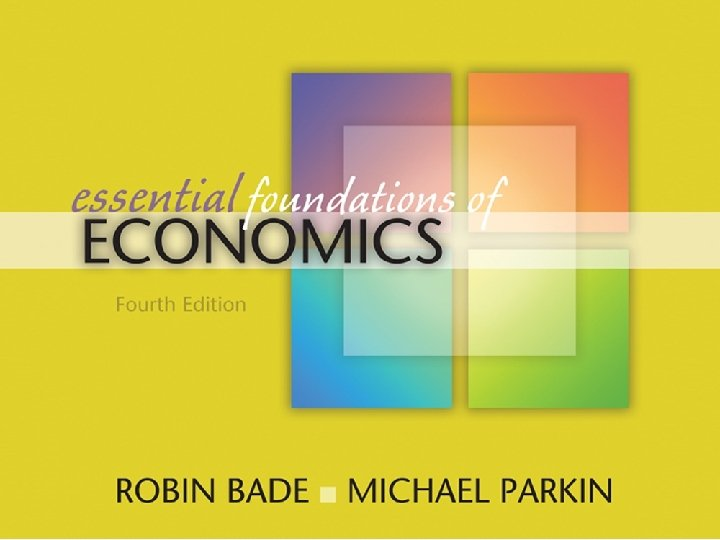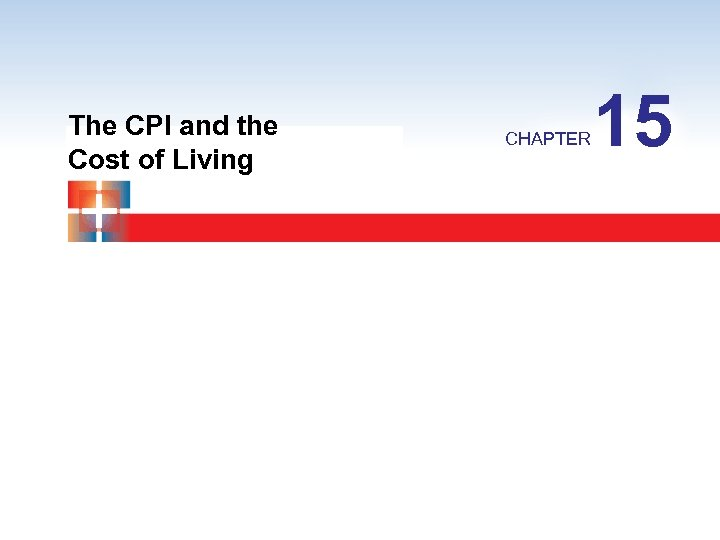The CPI and the Cost of Living CHAPTER 15CHAPTER CHECKLIST When you have completed your study of this chapter, you will be able to 1 Explain what the Consumer Price Index (CPI) is and how it is calculated. 2 Explain the limitations of the CPI and describe other measures of the price level. 3 Adjust money values for inflation and calculate real wage rates and real interest rates.15. 1 THE CONSUMER PRICE INDEX Consumer Price Index (CPI) is a measure of the average of the prices paid by urban consumers for a fixed market basket of consumer goods and services. The BLS calculates the CPI every month. We can use these numbers to compare what a fixed basket of goods costs this month with what it cost in some previous month.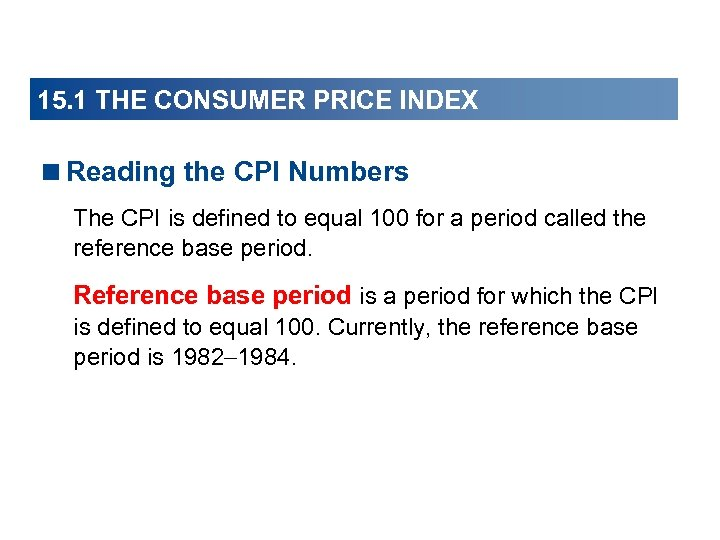15. 1 THE CONSUMER PRICE INDEX15. 1 THE CONSUMER PRICE INDEX In August 2007, the CPI was 207. 9. The average of the prices paid by urban consumers for a fixed market basket of consumer goods and services was 107. 9 percent higher in August 2007 than it was on the average during 1982 1984. In July 2007, the CPI was 208. 3. The average of the prices paid by urban consumers for a fixed market basket of consumer goods and services decreased by 0. 4 of a percentage point in August 2007.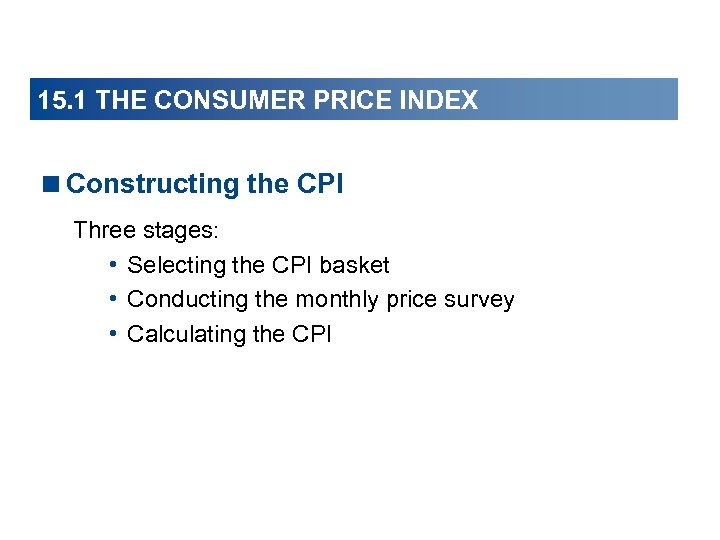15. 1 THE CONSUMER PRICE INDEX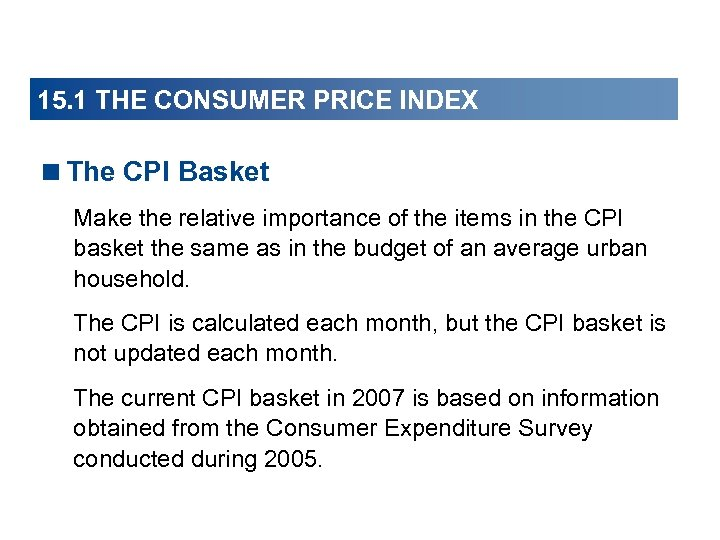15. 1 THE CONSUMER PRICE INDEX15. 1 THE CONSUMER PRICE INDEX Figure 15. 1 shows the CPI basket. This shopping cart is filled with the items that an average household buys.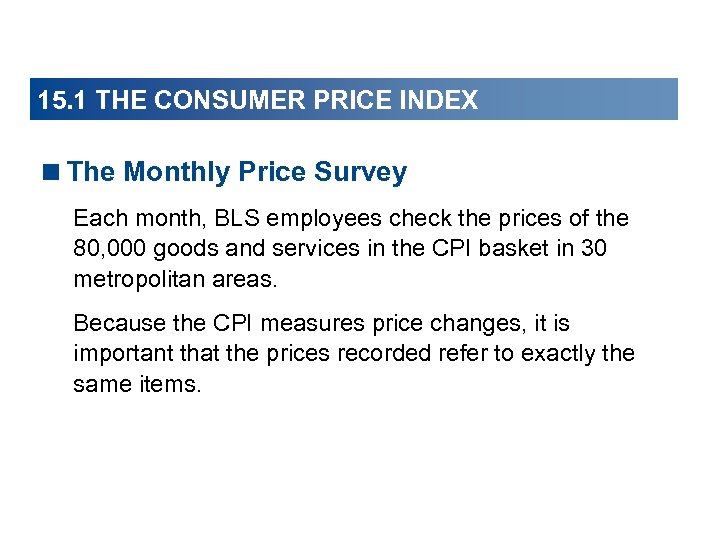15. 1 THE CONSUMER PRICE INDEX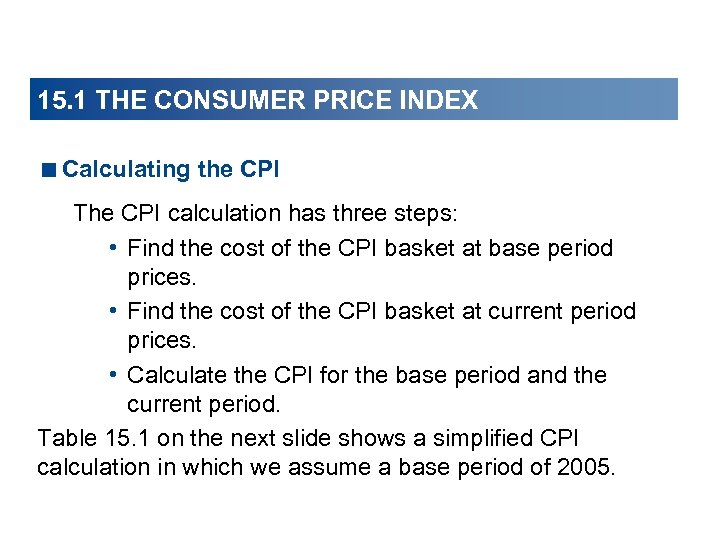15. 1 THE CONSUMER PRICE INDEX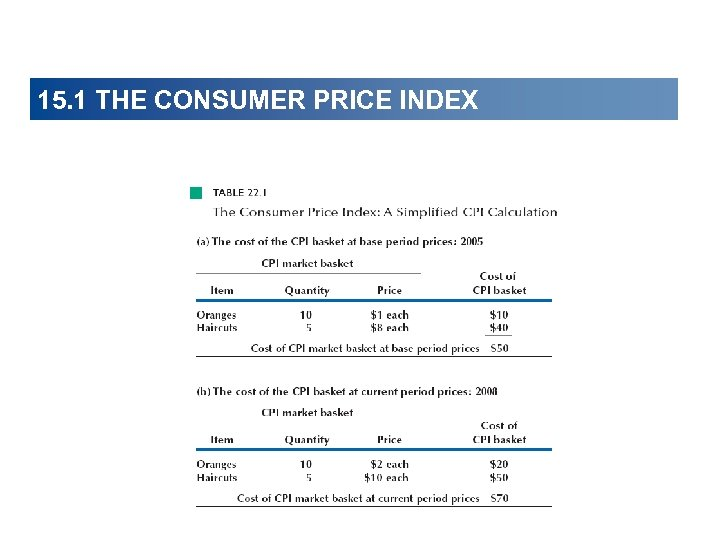15. 1 THE CONSUMER PRICE INDEX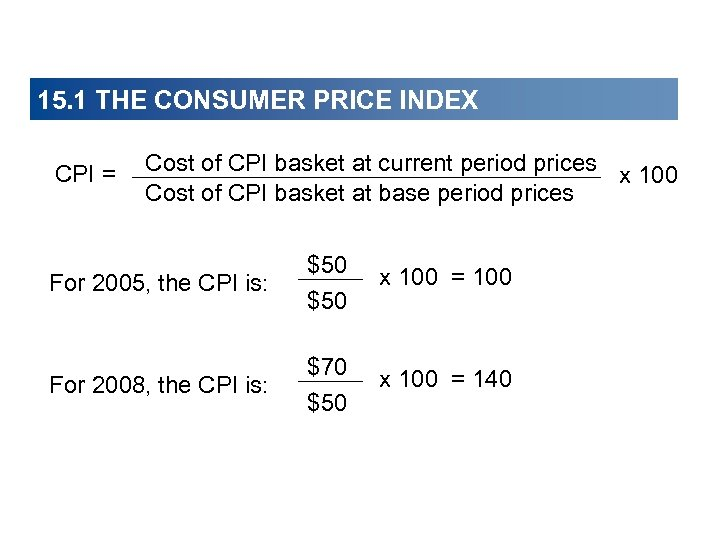15. 1 THE CONSUMER PRICE INDEX CPI = Cost of CPI basket at current period prices x 100 Cost of CPI basket at base period prices For 2005, the CPI is: \$50 x 100 = 100 For 2008, the CPI is: \$70 \$50 x 100 = 14015. 1 THE CONSUMER PRICE INDEX15. 1 THE CONSUMER PRICE INDEX Figure 15. 2 shows the CPI in part (a) and the inflation rate in part (b).15. 1 THE CONSUMER PRICE INDEX In part (a), the price level has increased every year. The rate of increase was rapid during the early 1980 s and slower during the 1990 s.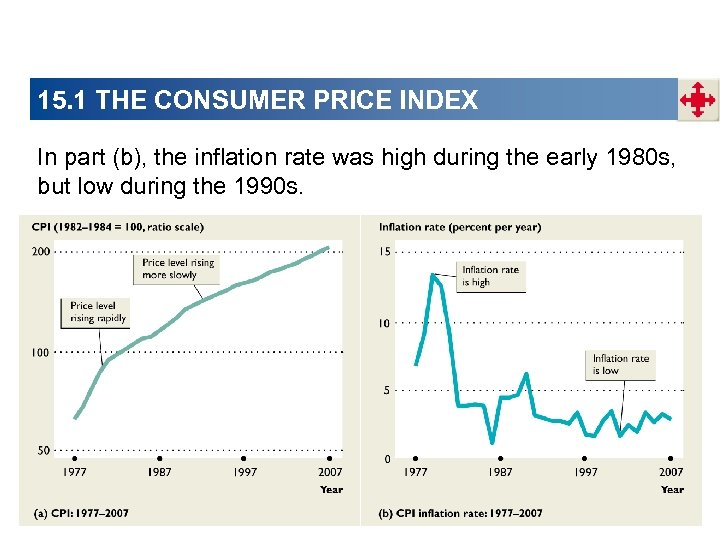15. 1 THE CONSUMER PRICE INDEX In part (b), the inflation rate was high during the early 1980 s, but low during the 1990 s.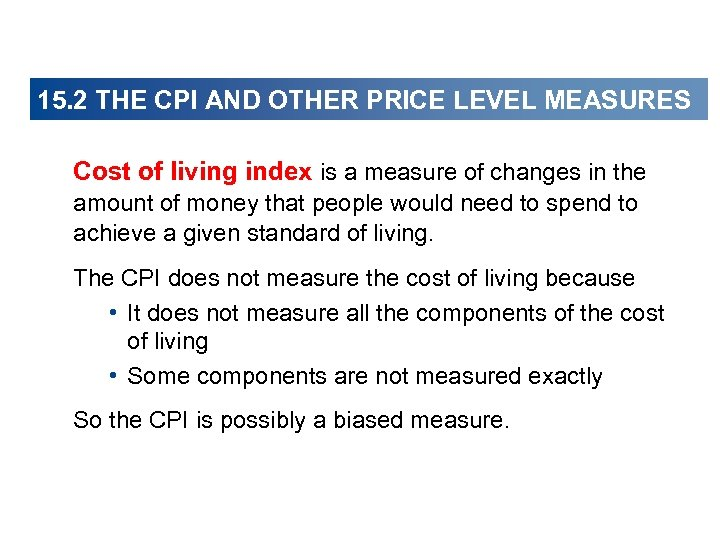15. 2 THE CPI AND OTHER PRICE LEVEL MEASURES Cost of living index is a measure of changes in the amount of money that people would need to spend to achieve a given standard of living. The CPI does not measure the cost of living because • It does not measure all the components of the cost of living • Some components are not measured exactly So the CPI is possibly a biased measure.15. 2 THE CPI AND OTHER PRICE LEVEL MEASURES < Sources of Bias in the CPI The potential sources of bias in the CPI are • New goods bias • Quality change bias • Commodity substitution bias • Outlet substitution bias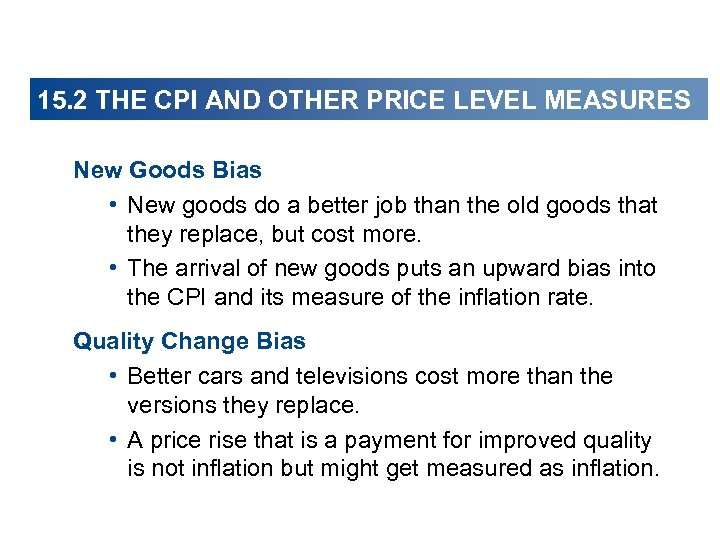15. 2 THE CPI AND OTHER PRICE LEVEL MEASURES New Goods Bias • New goods do a better job than the old goods that they replace, but cost more. • The arrival of new goods puts an upward bias into the CPI and its measure of the inflation rate. Quality Change Bias • Better cars and televisions cost more than the versions they replace. • A price rise that is a payment for improved quality is not inflation but might get measured as inflation.15. 2 THE CPI AND OTHER PRICE LEVEL MEASURES Commodity Substitution Bias • If the price of beef rises faster than the price of chicken, people buy more chicken and less beef. • The CPI basket doesn’t change to allow for the effects of substitution between goods. Outlet Substitution Bias • If prices rise more rapidly, people use discount stores more frequently. • The CPI basket doesn’t change to allow for the effects of outlet substitution.15. 2 THE CPI AND OTHER PRICE LEVEL MEASURES < The Magnitude of the Bias The Boskin Commission estimated the bias to be 1. 1 percentage points per year. If the measured inflation rate is 3. 1 percent a year, most likely the actual inflation rate is 2. 0 percent a year. To reduce the bias, the BLS has decided to increase the frequency of its Consumer Expenditure Survey and revise the CPI basket every two years. When the BLS revises the CPI basket, the reference base period does not change.15. 2 THE CPI AND OTHER PRICE LEVEL MEASURES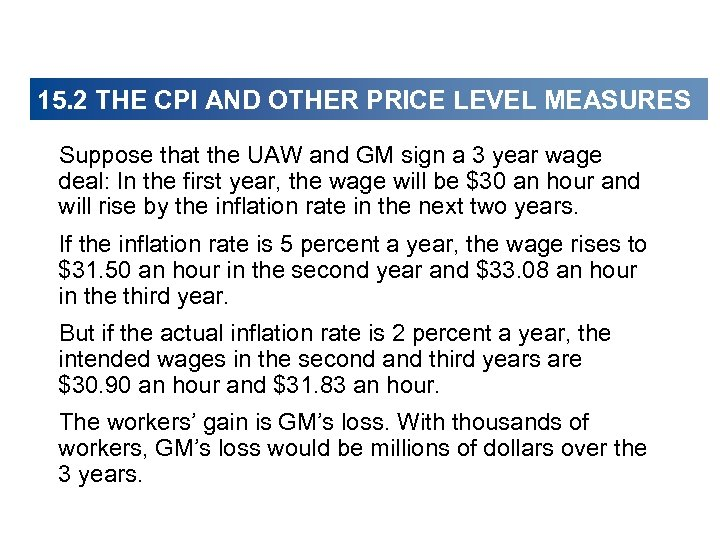15. 2 THE CPI AND OTHER PRICE LEVEL MEASURES Suppose that the UAW and GM sign a 3 year wage deal: In the first year, the wage will be \$30 an hour and will rise by the inflation rate in the next two years. If the inflation rate is 5 percent a year, the wage rises to \$31. 50 an hour in the second year and \$33. 08 an hour in the third year. But if the actual inflation rate is 2 percent a year, the intended wages in the second and third years are \$30. 90 an hour and \$31. 83 an hour. The workers’ gain is GM’s loss. With thousands of workers, GM’s loss would be millions of dollars over the 3 years.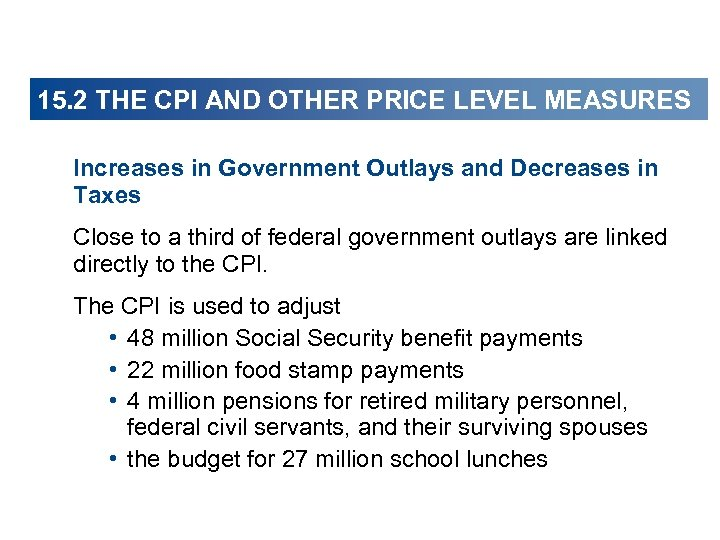15. 2 THE CPI AND OTHER PRICE LEVEL MEASURES Increases in Government Outlays and Decreases in Taxes Close to a third of federal government outlays are linked directly to the CPI. The CPI is used to adjust • 48 million Social Security benefit payments • 22 million food stamp payments • 4 million pensions for retired military personnel, federal civil servants, and their surviving spouses • the budget for 27 million school lunches15. 2 THE CPI AND OTHER PRICE LEVEL MEASURES The CPI is used to adjust the income levels at which higher tax rates apply. Because tax rates on large incomes are higher than those on small incomes as incomes rise, the burden of taxes would rise relentlessly if these adjustments were not made. To the extent that the CPI is biased upward, the tax adjustments over-compensate for rising prices and decrease the amount paid in taxes.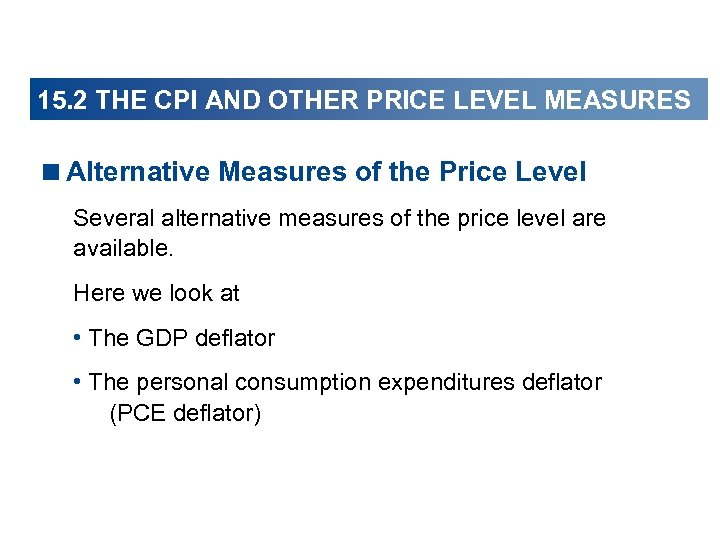15. 2 THE CPI AND OTHER PRICE LEVEL MEASURES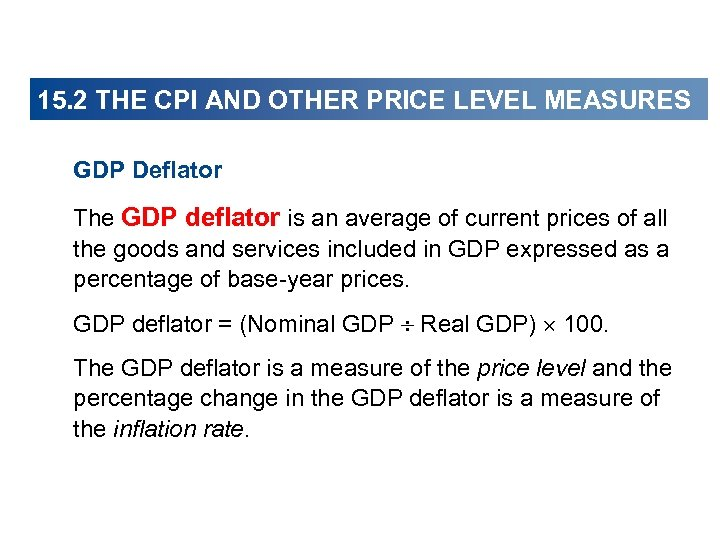15. 2 THE CPI AND OTHER PRICE LEVEL MEASURES GDP Deflator The GDP deflator is an average of current prices of all the goods and services included in GDP expressed as a percentage of base-year prices. GDP deflator = (Nominal GDP Real GDP) 100. The GDP deflator is a measure of the price level and the percentage change in the GDP deflator is a measure of the inflation rate.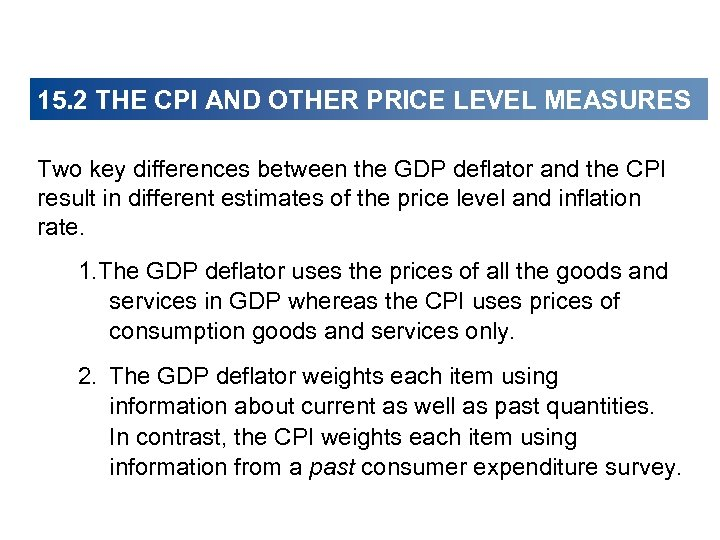15. 2 THE CPI AND OTHER PRICE LEVEL MEASURES Two key differences between the GDP deflator and the CPI result in different estimates of the price level and inflation rate. 1. The GDP deflator uses the prices of all the goods and services in GDP whereas the CPI uses prices of consumption goods and services only. 2. The GDP deflator weights each item using information about current as well as past quantities. In contrast, the CPI weights each item using information from a past consumer expenditure survey.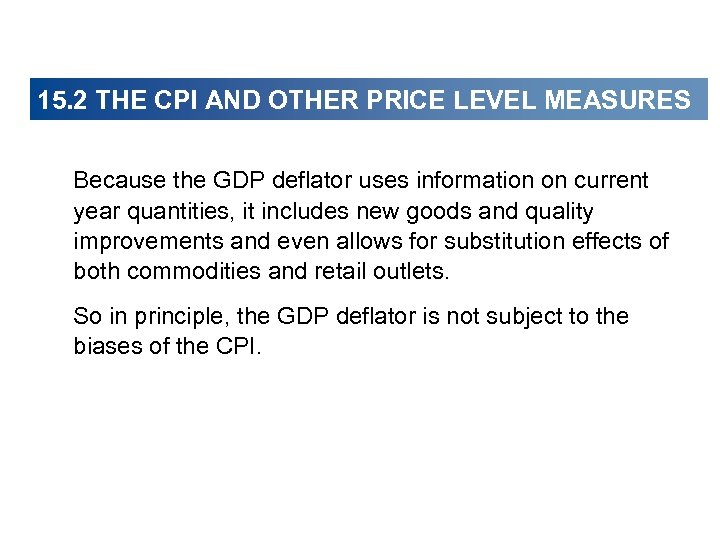15. 2 THE CPI AND OTHER PRICE LEVEL MEASURES Because the GDP deflator uses information on current year quantities, it includes new goods and quality improvements and even allows for substitution effects of both commodities and retail outlets. So in principle, the GDP deflator is not subject to the biases of the CPI.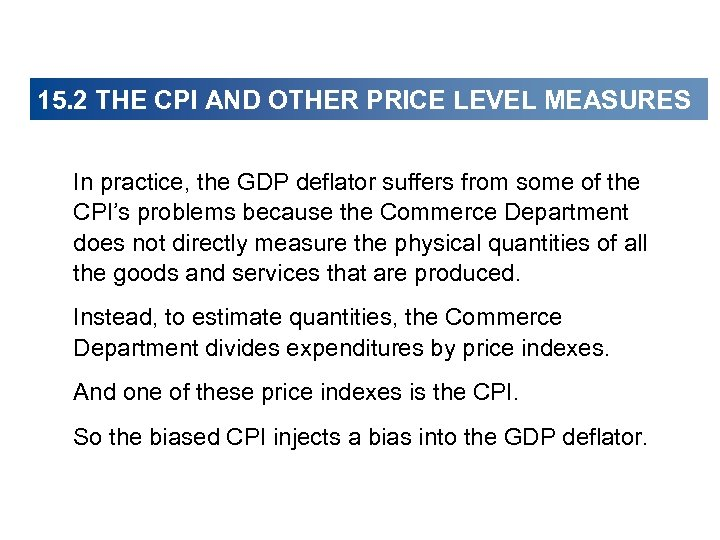15. 2 THE CPI AND OTHER PRICE LEVEL MEASURES In practice, the GDP deflator suffers from some of the CPI’s problems because the Commerce Department does not directly measure the physical quantities of all the goods and services that are produced. Instead, to estimate quantities, the Commerce Department divides expenditures by price indexes. And one of these price indexes is the CPI. So the biased CPI injects a bias into the GDP deflator.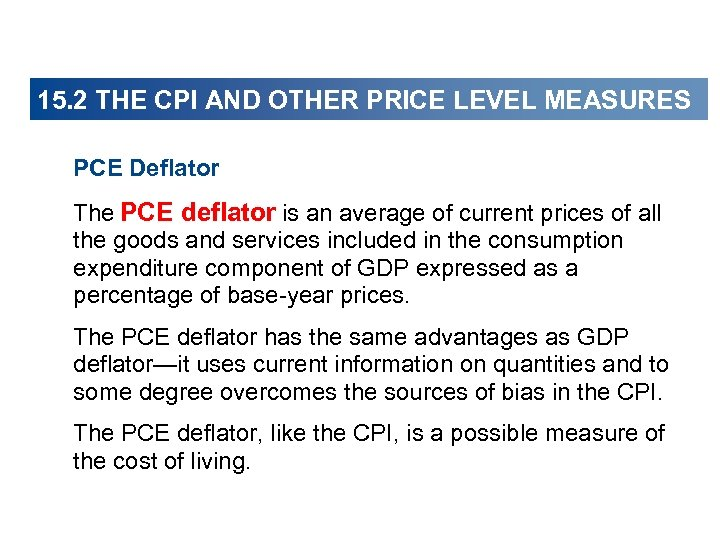15. 2 THE CPI AND OTHER PRICE LEVEL MEASURES PCE Deflator The PCE deflator is an average of current prices of all the goods and services included in the consumption expenditure component of GDP expressed as a percentage of base-year prices. The PCE deflator has the same advantages as GDP deflator—it uses current information on quantities and to some degree overcomes the sources of bias in the CPI. The PCE deflator, like the CPI, is a possible measure of the cost of living.15. 2 THE CPI AND OTHER… Figure 15. 3 shows the three measures of inflation in part (a) and the corresponding measures of the price level in part (b). The three measures of the inflation rate fluctuate together, but the CPI measure rises more rapidly than the GDP deflator measure or the PCE deflator measure.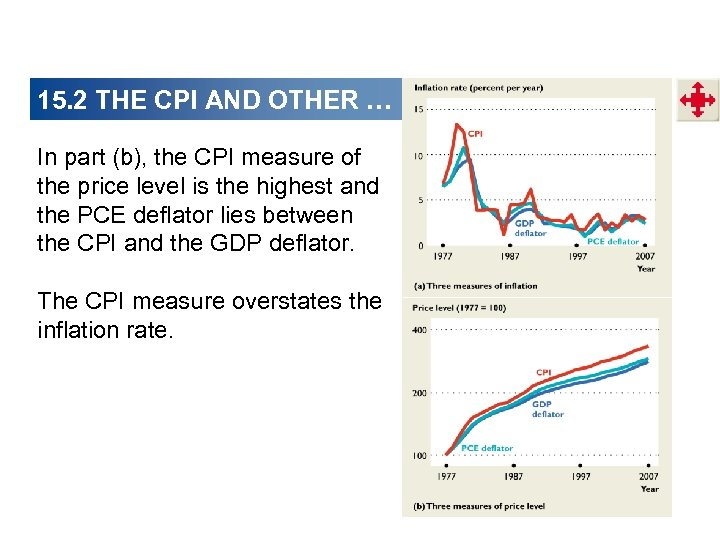15. 2 THE CPI AND OTHER … In part (b), the CPI measure of the price level is the highest and the PCE deflator lies between the CPI and the GDP deflator. The CPI measure overstates the inflation rate.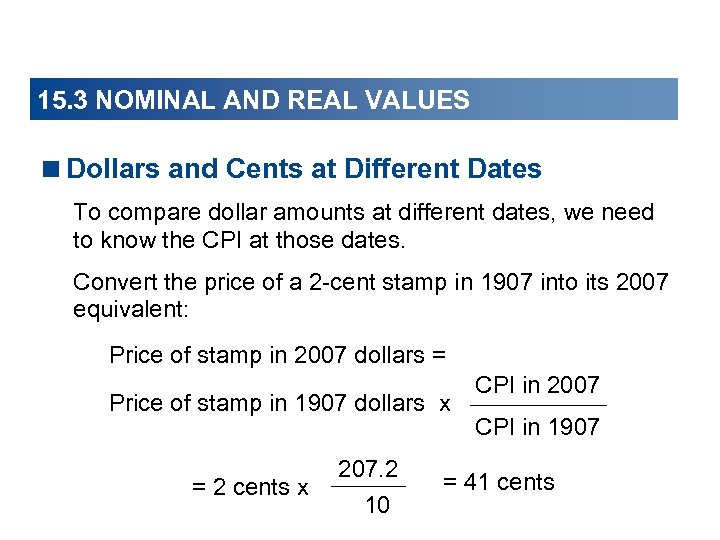15. 3 NOMINAL AND REAL VALUES15. 3 NOMINAL AND REAL VALUES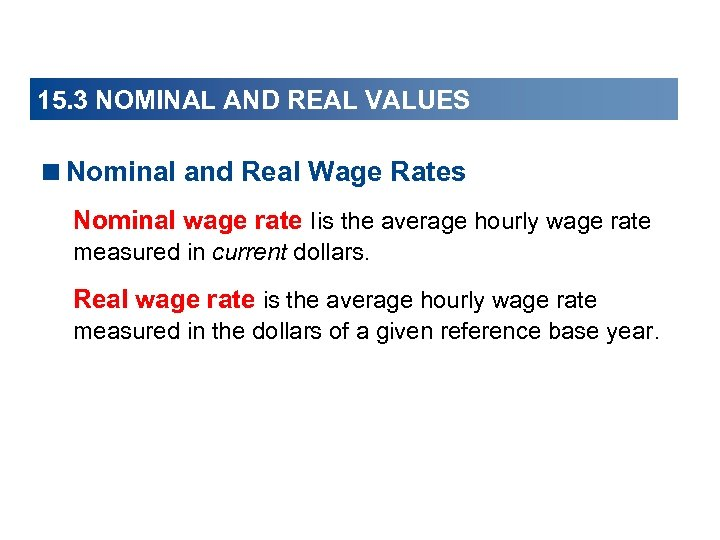15. 3 NOMINAL AND REAL VALUES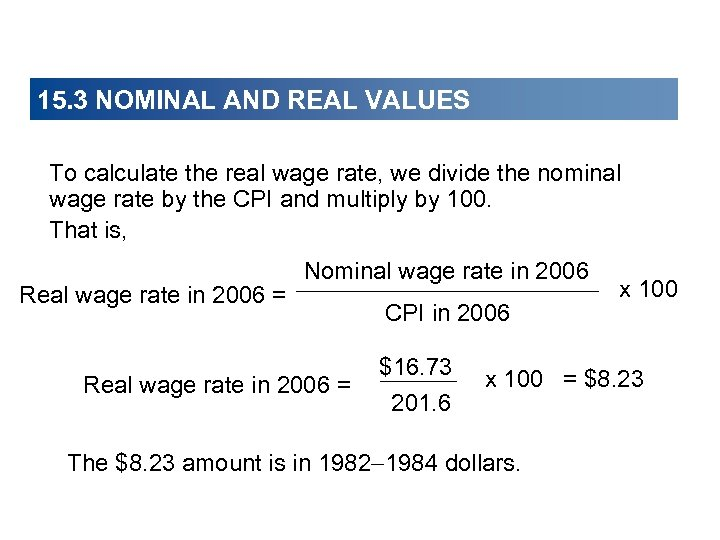15. 3 NOMINAL AND REAL VALUES To calculate the real wage rate, we divide the nominal wage rate by the CPI and multiply by 100. That is, Real wage rate in 2006 = Nominal wage rate in 2006 Real wage rate in 2006 = CPI in 2006 \$16. 73 201. 6 x 100 = \$8. 23 The \$8. 23 amount is in 1982 1984 dollars.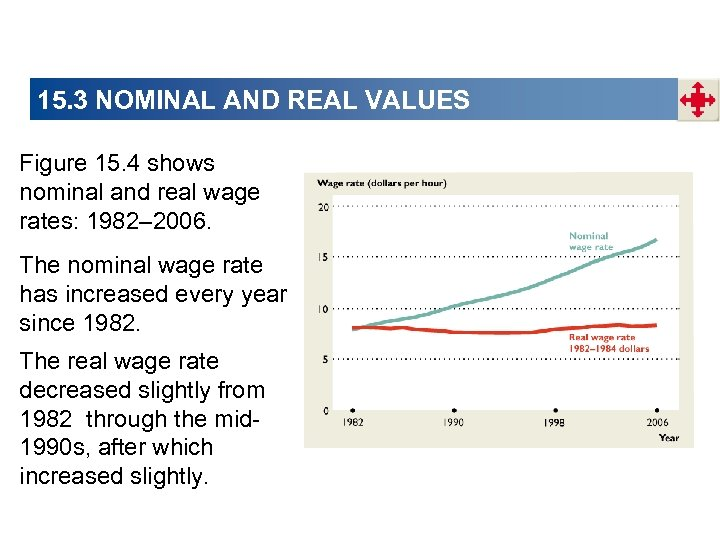15. 3 NOMINAL AND REAL VALUES Figure 15. 4 shows nominal and real wage rates: 1982– 2006. The nominal wage rate has increased every year since 1982. The real wage rate decreased slightly from 1982 through the mid 1990 s, after which increased slightly.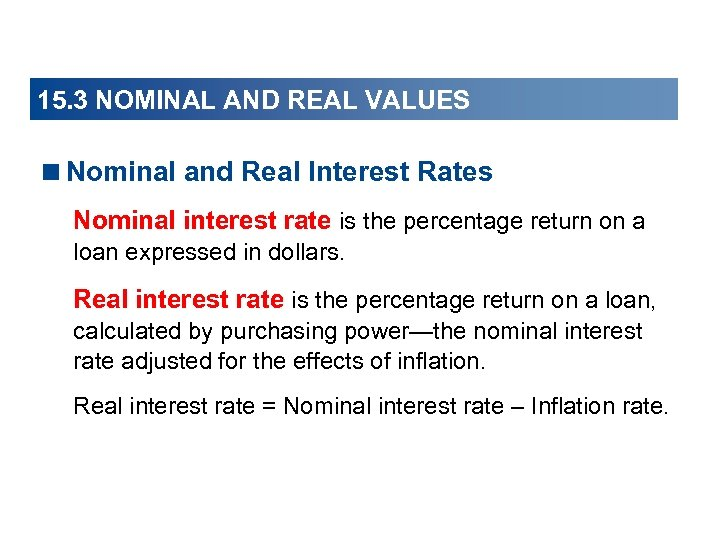15. 3 NOMINAL AND REAL VALUES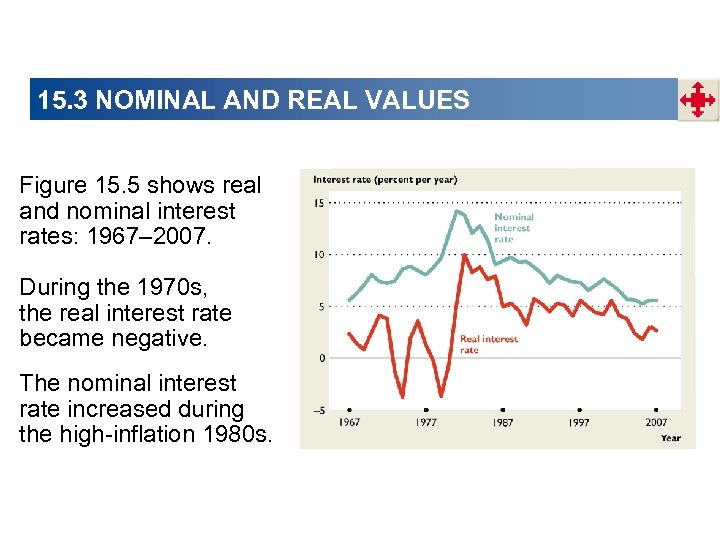15. 3 NOMINAL AND REAL VALUES Figure 15. 5 shows real and nominal interest rates: 1967– 2007. During the 1970 s, the real interest rate became negative. The nominal interest rate increased during the high-inflation 1980 s.